Students can Download Samacheer Kalvi 10th Maths Model Question Paper 3 English Medium Pdf, Samacheer Kalvi 10th Maths Model Question Papers helps you to revise the complete Tamilnadu State Board New Syllabus, helps students complete homework assignments and to score high marks in board exams.

## Tamil Nadu Samacheer Kalvi 10th Maths Model Question Paper 3 English Medium

Instructions

• The question paper comprises of four parts.
• You are to attempt all the parts. An internal choice of questions is provided wherever applicable.
• All questions of Part I, II, III and IV are to be attempted separately.
• Question numbers 1 to 14 in Part I are Multiple Choice Quèstions of one-mark each. These are to be answered by choosing the most suitable answer from the given four alternatives and.writing the option code and the corresponding answer.
• Question numbers 15 to 28 in Part II àre two-marks questions. These are to be answered in about one or two sentences.
• Question numbers 29 to 42 in Part III are five-marks questions. These are to be answered in about three to five short sentences.
• Question numbers 43 to 44 in Part IV are eight-marks questions. These are to be answered in detail. Draw diagrams wherever necessary.

Time: 3 Hours
Maximum Marks: 100

PART – I

I. Choose the correct answer. Answer all the questions. [14 × 1 = 14]

Question 1.
If the ordered pairs (a + 2, 4) and (5, 2a + b) are equal then (a, b) is …………. .
(1) (2,-2)
(2) (5,1)
(3) (2,3)
(4) (3,-2)
(4) (3,-2)Question 2.
f(x) = (x + 1)3 – (x – 1)3 represents a function which is …………. .
(1) linear
(2) cubic
(3) reciprocal

Question 3.
Using Euclid’s division lemma, if the cube of any positive integer is divided by 9 then the possible remainders are …………. .
(1) 0,1,8
(2) 1,4,8
(3) 0,1,3
(4) 1,3,5
(1) 0,1,8

Question 4.
If the sequence t1, t2, t3, are in A.P. then the sequence t6, t12, t18, …….. is …………….
(1) a Geometric progression
(2) an Arithmetic progression
(3) neither an Arithmetic progression nor a Geometric progression
(4) a constant sequence
(2) an Arithmetic progression

Question 5.
$$\frac{3 y-3}{y} \div \frac{7 y-7}{3 y^{2}}$$ is ………….
(1) $$\frac{9 y}{7}$$
(2) $$\frac{9 y^{3}}{(21 y-21)}$$
(3) $$\frac{21 y^{2}-42 y+21}{3 y^{3}}$$
(4) $$\frac{7\left(y^{2}-2 y+1\right)}{y^{2}}$$
$$\frac{9 y}{7}$$

Question 6.
The solution of (2x – 1)2 = 9 is equal to …………. .
(1) -1
(2) 2
(3) -1,2
(4) None of these
(3) -1,2

Question 7.
In ∆LMN, ∠L = 60°, ∠M = 50°. If ∆LMN ~ ∆PQR then the value of ∠R is …………. .
(1) 40°
(2) 70°
(3) 30°
(4) 110°
(2) 70°

Question 8.
A tower is 60 m height. Its shadow is x metres shorter when the sun’s altitude is 45° than when it has been 30° , then x is equal to…………. .
(1) 41.92 m
(2) 43.92 m
(3) 43 m
(4) 45.6 m
(2) 43.92 mQuestion 9.
A shuttle cock used for playing badminton has the shape of the combination of …………. .
(1) a cylinder and a sphere
(2) a hemisphere and a cone
(3) a sphere and a cone
(4) frustum of a cone and a hemisphere
(4) frustum of a cone and a hemisphere

Question 10.
Which of the following is not a measure of dispersion?
(1) Range
(2) Standard deviation
(3) Arithmetic mean
(4) Variance
(3) Arithmetic mean

Question 11.
Variance of first 20 natural numbers is …………. .
(1) 32.25
(2) 44.25
(3) 33.25
(4) 30
(3) 33.25

Question 12.
The equation of a straight line having slope 3 and Y intercept -4 is …………. .
(1) 3x – y + 4 = 0
(2) 3x + y – 4 = 0
(3) 3x – y + 4 = 0
(4) 3x – y – 4 = 0
(4) 3x – y – 4 = 0

Question 13.
In the given diagram PA and PB are tangents drawn from P to a circle with centre O ∠OPA = 35° then a and b is …………. .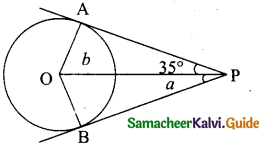(1) a = 30°, b = 60°
(2) a = 35°, b = 55°
(3) a = 40°, b = 50°
(4) a = 45°, b = 45°
(2) a = 35°, b = 55°

Question 14.
If $$\left[ \begin{matrix} -1 & -2 & 4 \end{matrix} \right] \left[ \begin{matrix} 2 \\ a \\ -3 \end{matrix} \right] = -10$$ then the value of “a” is …………. .
(1) 2
(2) -4
(3) 4
(4) -2
(4) -2PART – II

II. Answer any ten questions. Question No. 28 is compulsory. [10 × 2 = 20]

Question 15.
Show that the function f : N → N defined by f(m) = m2 + m + 3 is one-one function.
N = {1,2, 3,4, 5,………. }
f(m) = m2 + m + 3
f(1) = 12 + 1 + 3 = 5
f(2) = 22+ 2 + 3 = 9
f(3) = 32 + 3 + 3 = 15
f(4) = 42 + 4 + 3 = 23
f = {(1,5) (2, 9) (3, 15) (4, 23)}
From the diagram we can understand different elements in (N) in domain,
there are different images in (N) co-domain.
∴ The function is one-one function.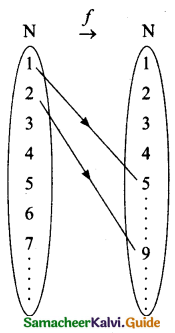Question 16.
If the ordered pairs (x2 – 3x, y2 + 4y) and (-2, 5) are equal, then find x and y.
(x2 – 3x, y2 + 4y) = (-2, 5)
x2 – 3x = -2
x2 – 3x + 2 = 0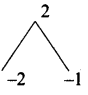(x – 2)(x – 1) = 0
x – 2 = 0 or x – 1 =0
x = 2 or 1

y2 + 4y = 5
y2 + 4y – 5 = o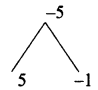(y + 5)(y – 1) = o
y + 5 = 0 or y – 1 = 0
y = -5 or y = 1Question 17.
If x is congruent to 13 modulo 17 then 7x – 3 is congruent to which number modulo 17?
Given x ≡ 13 (mod 17) ……. (1)
7x – 3 ≡ a (mod 17) ….(2)
From (1) we get
x – 13 = 17 n(n may be any integer)
x – 13 is a multiple of 17
∴ The least value of x = 30
From (2) we get
7(30) – 3 ≡ a(mod 17)
210 – 3 ≡ a(mod 17)
207 ≡ a(mod 17)
207 ≡ 3(mod 17)
∴ The value of a = 3

Question 18.
How many terms of the series 1 + 4 + 16 + . . . . make the sum 1365?
Let n be the number of terms to be added to get the sum 1365
a = 1, r = $$\frac { 4 }{ 1 }$$ = 4 > 1
sn = 1365 gives $$\frac{a\left(r^{n}-1\right)}{r-1}$$ = 1365
$$\frac{1\left(4^{n}-1\right)}{4-1}$$ = 1365 so, (4n – 1) = 4095
4n = 4096 then 4n = 46
n = 6

Question 19.
Find the LCM of x4 – 27a3x, (x – 3a)2 whose GCD is (x – 3a)
p(x) = x4 – 27a3x
= x[x3 – 27a3]
= x[x3 – (3a)3]
= x(x – 3a) (x2 + 3ax + 9a2)
g(x) = (x – 3a)2
G.C.D. = x – 3a
L.C.M = $$\frac{p(x) \times g(x)}{\text { G.C.D. }}$$
= $$\frac{x(x-3 a)\left(x^{2}+3 a x+9 a^{2}\right) \times(x-3 a)^{2}}{(x-3 a)}$$
L.C.M = x(x – 3a)2(x2 + 3ax + 9a2)

Question 20.
Reduce the given Rational expression to its lowest form $$\frac{x^{3 a}-8}{x^{2 a}+2 x^{a}+4}$$
x3a – 8 = (xa)3 – 23
= (xa – 2) [(xa)2 + xa × 2 + 22]
= (xa – 2) (x2a + 2xa + 4)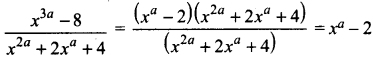Question 21.
Find the values of x,y, z if (x y -z z + 3) + (y 4 3) = (4 8 16)
[x y – z z + 3] + [y 4 3] = [4 8 16]
x + y = 4 ….(1)
y – z + 4 = 8 ….(2)
z + 3 + 3 = 16
z + 6 = 16
z = 16 – 6 = 10
Sùbstitute the value of z in (2)
(2) ⇒ y – 10 = 4 ⇒ y = 14
Substitute the value of y in (1)
(1) ⇒ x + 14 = 4
x = 4 – 14 = -10
∴ The value of x = -10, y = 14 and z = 10

Question 22.
Suppose AB, AC and BC have lengths 13,14 and 15 respectively. If $$\frac{A F}{F B}=\frac{2}{5}$$ and $$\frac{C E}{E A}=\frac{5}{8}$$ Find BD and DC.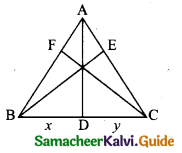Given that AB = 13, AC = 14 and BC = 15
Let BD = x and DC = y
Using Ceva’s theorem, we have, $$\frac{\mathrm{BD}}{\mathrm{DC}} \times \frac{\mathrm{CE}}{\mathrm{EA}} \times \frac{\mathrm{AF}}{\mathrm{FB}}=1$$ ….(1)
Substitute the values of $$\frac{A F}{F B}$$ and $$\frac{C E}{E A}$$ in (1),
we have $$\frac{B D}{D C} \times \frac{5}{8} \times \frac{2}{5}=1$$
$$\frac{x}{y} \times \frac{10}{40}=1$$ we get $$\frac{x}{y} \times \frac{1}{4}=1$$. Hence, x = 4y ……. (2)
BC = BD + DC = 15 so, x + y = 15 ……. (3)
From (2), using x = 4y in (3) we get, 4y + y = 15 gives 5y = 15 then y = 3
Substitute y = 3 in (3) we get, x = 12. Hence BD = 12 , DC = 3.

Question 23.
Find the equation of a straight line which has slope $$\frac { -5 }{ 4 }$$ and passing through the point (-1,2).
Slope of a line (m) = $$\frac{-5}{4}$$
The given point (x1, y1) = (-1, 2)
Equation of a line is y – y1 = m (x – x1)
y – 2 = $$\frac{-5}{4}$$(x + 1)
5(x + 1) = 4(y – 2) ⇒ 5x + 5 = 4y + 8
5x + 4y + 5 – 8 = 0 ⇒ 5x + 4y – 3 = 0
The equation of a line is 5x + 4y – 3 = 0Question 24.
A road is flanked on either side by continuous rows of houses of height 4√3 m with no space in between them. A pedestrian is standing on the median of the road facing a row house. The angle of elevation from the pedestrian to the top of the house is 30° . Find the width of the road.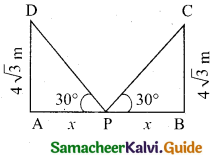Let the mid point of the road AB is “P” (PA = PB)
Height of the home = 4 √3 m
Let the distance between the pedestrian and the house be “x”
In the right ∆ APD, tan 30° = $$\frac{\mathrm{AD}}{\mathrm{AP}}$$
$$\frac{1}{\sqrt{3}}=\frac{4 \sqrt{3}}{x}$$
x = 4√3 × √3 = 12 m
∴ Width of the road = PA + PB
= 12 + 12
= 24m

Question 25.
If the standard deviation of a data is 3.6 and each value of the data is divided by 3, then find the new variance and new standard deviation.
Standard deviation of the data = 3.6
Each value of the data is divided by 3
New standard deviation = $$\frac{3.6}{3}$$ = 1.2
New Variance = (1.2)2 [Variance = (S. D)2]
= 1.44
New standard Deviation = 1.2
New variance = 1.44

Question 26.
If -4 is a root of the equation x2 + px – 4 = 0 and if the equation x2 + px + q = 0 has equal roots, find the values of p and q.
Let p(x) = x2 + px – 4
-4 is the root of the equation
p(-4)  = 0
16 – 4p – 4 = 0 ⇒ -4p + 12 = 0
-4p = -12
p = $$\frac { 12 }{ 4 }$$ = 3
The equation x2 + px + q = 0 has equal roots x2 + 3x – q = O
Here a = 1,b = 3,c = q
since the roots are real and equal
b2 – 4ac = 0 ⇒ 32 – 4(1) (q) = 0
9 – 4q = 0 ⇒ 94q
q = $$\frac{9}{4}$$
∴ The value of p = 3 and q = $$\frac{9}{4}$$Question 27.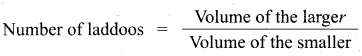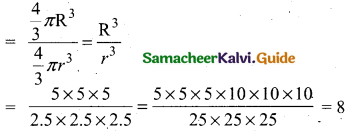Question 28.
If P(A) = $$\frac { 1 }{ 2 }$$ ; P(B) = $$\frac { 7 }{ 10 }$$ , P(A ∪ B) = 1 find P(A’ ∩ B’)
Given P(A) = $$\frac { 1 }{ 2 }$$, P(B) = $$\frac { 7 }{ 10 }$$, P(A ∪ B) = 1
P(A ∪ B) = P(A) + P(B) – P(A ∩ B)
1 = $$\frac{1}{2}+\frac{7}{10}$$ – P(A ∩ B)
P(A ∩ B) = $$\frac{1}{2}+\frac{7}{10}-1=\frac{5+7-10}{10}=\frac{2}{10}=\frac{1}{5}$$
P(A’ ∪ B’) = P(A ∩ B)’
= 1 – P(A ∩ B)
= $$1-\frac{1}{5}=\frac{5-1}{5}=\frac{4}{5}$$

PART – III

III. Answer any ten questions. Question No. 42 is compulsory. [10 × 5 = 50]

Question 29.
If X = {-5, 1, 3, 4} and Y = {a, b, c}, then which of the following relations are functions from X to Y?
(i) R1 = {(-5, a), (1, a), (3, b)}
(ii) R2 = {(-5, b), (1, b), (3, a), (4, C)}
(iii) R3 = {(-5, a), (1, a), (3, b), (4, c), (1, b)}

Question 30.
If f(x) = x2, g(x) = 3x and h(x) = x – 2, Prove that (fog)oh = fo(g o h).

Question 31.
The houses of a street are numbered from 1 to 49. Senthil’s house is numbered such that the sum of numbers of the houses prior to Senthil’s house is equal to the sum of numbers of the houses following Senthil’s house. Find Senthil’s house number.

Question 32.
The 104th term and 4th term of an A.P are 125 and 0. Find the sum of first 35 terms.

Question 33.
There are 12 pieces of five, ten and twenty rupee currencies whose total value is ₹105. But when first 2 sorts are interchanged in their numbers its value will be increased by ₹20. Find the number of currencies in each sort.

Question 34.
If 9x4 + 12x3 + 28x2 + ax + b is a perfect square, find the values of a and b.

Question 35.
Find X and Y if X + Y = $$\left( \begin{matrix} 7 & 0 \\ 3 & 5 \end{matrix} \right)$$ and X – Y = $$\left( \begin{matrix} 3 & 0 \\ 0 & 4 \end{matrix} \right)$$Question 36.
In figure ABC is a triangle with ∠B = 90° , BC = 3 cm and AB = 4 cm. D is point on AC such that AD = 1 cm and E is the midpoint of AB. Join D and E and extend DE to meet CB at F. Find BF.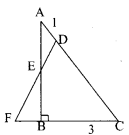Question 37.
Find the equation of a straight line joining the point of intersection of 3x + y + 2 = 0 and x – 2y -4 = 0 to the point of intersection of 7x – 3y = -12 and 2y = x + 3

Question 38.
A man is standing on the deck of a ship, which is 40 m above water level. He observes the angle of elevation of the top of a hill as 60° and the angle of depression of the base of the hill as 30° . Calculate the distance of the hill from the ship and the height of the hill. (√3 = 1.732)

Question 39.
Find the number of coins, 1.5 cm in diameter and 2 mm thick, to be melted to form a right circular cylinder of height 10 cm and diameter 4.5 cm.

Question 40.
The following table gives the number of goals scored by 71 leading players in international football matches. Find the standard deviation of the data.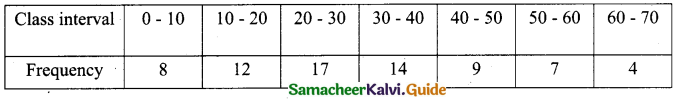Question 41.
A two digit number is such that the product of its digits is 18. When 63 is subtracted from the number, the digits interchange their places. Find the numbers.

Question 42.
The sum of 5th and 9th term of an AP is 72 and the sum of 7th and 12th term is 97. Find the A.P.PART – IV

IV. Answer all the questions. [2 × 8 = 16]

Question 43.
(a) Construct a triangle similar to a given triangle LMN with its sides equal to $$\frac { 4 }{ 5 }$$ of the corresponding sides of the triangle LMN (scale factor $$\frac { 4 }{ 5 }$$).

[OR]

(b) Draw the two tangents from a point which is 10 cm away from the centre of a circle of radius 5 cm. Also, measure the lengths of the tangents.

Question 44.
(a) Draw the graph of y = x2 – 4 and hence solve x2 – x – 12 = 0.

[OR]

(b) Solve graphically (2x + 1) (x -3) = 0